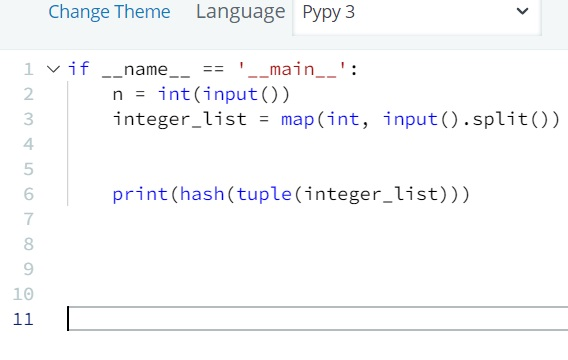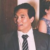# Hackerrank – Python – Basic Data Types – Tuples (Solution)

Publish Date - February 5th, 2023

|

A fun little problem with Tuples where you shouldn’t over think the execution of this. Less code is better! Note, I’ve noticed that nothing actually works in Python 3, so stick to PyPy3 instead for this question. While this isn’t harder than any particular SQL, Java or JavaScript problem – it can be annoying trying to get the interface to work

## The Problem

Given an integer, , and  space-separated integers as input, create a tuple, , of those  integers. Then compute and print the result of .

Note: hash() is one of the functions in the `__builtins__` module, so it need not be imported.

Input Format

The first line contains an integer, , denoting the number of elements in the tuple.
The second line contains  space-separated integers describing the elements in tuple .

Output Format

Print the result of .

Sample Input 0

```2
1 2
```

Sample Output 0

`3713081631934410656`

## The Solution

``````if __name__ == '__main__':
n = int(input())
integer_list = map(int, input().split())

print(hash(tuple(integer_list)))
``````

Simple solution, with you just printing out the tuple(integer_list).

Pretty much the only usage of code was the print function and tuple function, both are which handle single values. The mistake I made was thinking I needed to use LIST comprehension or something to loop through all of the values of the tuple (which was a wrong thought).

While this may not be a machine learning or NLP problem, understanding the basics are important!

### 8 thoughts on “Hackerrank – Python – Basic Data Types – Tuples (Solution)”

1.yeah you should Switch to PyPy3 it Worked..!

2.this code giving output to me (-3550055125485641917)
and it show error

1.Hi Riya,

What input are you doing!

I just submitted my answer again it worked.

Try doing the solution in PyPy3

1.hello me too i got the same result (-3550055125485641917)
i didn’t understand what the problem is ?

2.Naveen Pandit

Hey,
I also got the same output.
Did you find any error

1.Yes – switch out of “Python 3” and into PyPy3!

1.what the solution for python 3 not for pypy3?